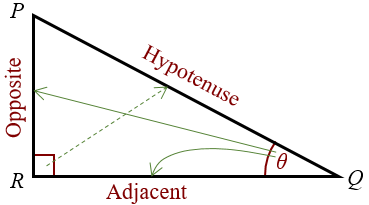# Precalculus

Formula Sheet

Make sure to focus on logarithm, polynomial, and exponential functions. In addition, work on synthetic division and analytical geometry. Lastly, learn about sigma notation, sequences/sums, and proof by induction.

### Stuff to remember:

#### Trig:

pi = 180 degrees

Soh Cah Toa

Sin = Opposite/Hypotenuse

##### Reciprocals

Csc = Hypotenuse/Opposite or 1/Sin

Cot = Adjacent/Opposite (= cos/sin) or 1/Tan

##### Inverse Trigonometric Functions

Note that using the power of -1 here is not actually raising it to the -1 power, but rather a notation often used for inverse functions that may appear on your calculator.

tan^-1 aka (arctan) is the inverse function for tangent

cos^-1 aka (arccos) is the inverse function for cosine

sin^-1 aka (arcsin) is the inverse function for sine

Application: As sin(pi/2) = 1, arcsin(1) = pi/2

These come in handy for calculus

##### Functions

One to One = Injective

Onto = Surjective

Both = Bijective

###### Vertical line test:

For a graph to represent a function, it would need to have 1 inout for each output, IE any vertical line would need to hit the graph at most once. Using this test, we know the equation of a circle isn’t considered to be a function.

###### Horizontal line test:

Use the horizontal line test to see if it is surjective, injective, bijective, or none. For a function to be one to one, the graph of the function crosses any horizontal line on the graph at least once. Whereas, for onto it would need to cross at most once. For it to be both onto and one to one, the graph would need to cross any horizontal line at most one time.##### Resources

My formula sheet

Exponent Rules

Polynomial Inequalities

Polynomial Division

Domain, Range, and Signs of Trigonometric Functions

Updated on July 6, 2023